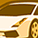PSoC™ 5, 3 & 1Level 2Level 2

# problems with gyroscope angle with MPU6050

hello ,

I have some problems with getting the angle from the gyroscope of the MPU6050. I am doing the following:

``````GyroX0 = GyroX; // previous Gyro value
GyroY0 = GyroY; // previous Gyro value
GyroZ0 = GyroZ; // previous Gyro value

GyroX = mybuf/131.07; // Current Gyro value
GyroY = mybuf/131.07;// Current Gyro value
GyroZ = mybuf/131.07; // Current Gyro value

GyroX = GyroX - GyroErrorX; // Current gyro - error value from MPU6050
GyroY = GyroY - GyroErrorY; // Current gyro - error value from MPU6050
GyroZ = GyroZ - GyroErrorZ; // Current gyro - error value from MPU6050

GyroRateX = GyroX - GyroX0; // get rate of change by Current Gyro - old Gyro
GyroRateY = GyroY - GyroY0; // get rate of change by Current Gyro - old Gyro
GyroRateZ = GyroZ - GyroZ0; // get rate of change by Current Gyro - old Gyro

//Delta time is ms =>  ms /1000 = s
gyroAngleX = gyroAngleX + GyroRateX * (DeltaTime/1000.0);
gyroAngleY = gyroAngleY + GyroRateY * (DeltaTime/1000.0);
gyroAngleZ = gyroAngleZ + GyroRateZ * (DeltaTime/1000.0);    ``````

The function for deltatime you can find here.  The full project is also avaible is the fille attached .

Can someone help??

1 SolutionModeratorModerator

# Re: problems with gyroscope angle with MPU6050

Hi @Switten,

Can you please let me know what is the exact issue you are facing?

1) From my experience, there might be an issue with the calibration of the sensor. This is the link to the GitHub project for how to calibrate the sensor. I have referred to this and calibrated the BMI 160 sensor. Find out the offset and subtract it from raw data.

2) You can refer to this project for the Angle calculation using a complementary filter. For accelpitch and accelroll I have used different equations than given in the project.

accelRoll  = (atan2(-AY, AZ)*180.0)/M_PI;

accelPitch = (atan2(AX, sqrt(AY*AY + AZ*AZ))*180.0)/M_PI;

(

Where,                    AX = ((float)CAX-AXoff)/16384.00;

AY = ((float)CAY-AYoff)/16384.00;

AZ = ((float)CAZ-(AZoff-16384))/16384.00;

)

3) Can you please check the sensitivity configuration for a gyro. Link

Please, go through the above projects and try to implement them. Let us know if further clarification is required.

Thank you

Best Regards

Raj Chaudhari

4 RepliesModeratorModerator

# Re: problems with gyroscope angle with MPU6050

Hi @Switten,

Can you please let me know what is the exact issue you are facing?

1) From my experience, there might be an issue with the calibration of the sensor. This is the link to the GitHub project for how to calibrate the sensor. I have referred to this and calibrated the BMI 160 sensor. Find out the offset and subtract it from raw data.

2) You can refer to this project for the Angle calculation using a complementary filter. For accelpitch and accelroll I have used different equations than given in the project.

accelRoll  = (atan2(-AY, AZ)*180.0)/M_PI;

accelPitch = (atan2(AX, sqrt(AY*AY + AZ*AZ))*180.0)/M_PI;

(

Where,                    AX = ((float)CAX-AXoff)/16384.00;

AY = ((float)CAY-AYoff)/16384.00;

AZ = ((float)CAZ-(AZoff-16384))/16384.00;

)

3) Can you please check the sensitivity configuration for a gyro. Link

Please, go through the above projects and try to implement them. Let us know if further clarification is required.

Thank you

Best Regards

Raj ChaudhariLevel 2Level 2

# Re: problems with gyroscope angle with MPU6050

hi there @Raj_JC

``GyroX = GyroX - GyroErrorX``

Here is the Gyro Error the offset value after 200 data readings.

Solution 2 was not necessary because the accelerometer is already working en giving the correct angle.

For the last (solution 3) thank you for this, had forgotten that my MPU had stil default calibrations, so this has been adjusted.

But even thought I did this I still don't get a accurate gyro-angle. I have added a video that gives more information. (B.e. If you look at the video you will see that afer 3 seconds you will see that the gyro read a angle of  -50 & -68 while I just moved the MPU 90° around the Y axis.

Also the original project file is also updated to the most current one. See attached file in the question.

Best regards

SwittenModeratorModerator

# Re: problems with gyroscope angle with MPU6050

Hi @Switten,

You can try the following things to debug:

1) Try to calculate angle without a complementary filter. Calculate individual angles by gyro and accelerometer. Check if they are getting calculated correctly. If yes then combine them.

2) Time delay is 0.5 s, which can also cause the issue. Even in my implementation when I try to increase the time delay for consecutive readings the angle sensed by gyro is wrong. Please, try to reduce the time delay and verify if it improves the angle sensing.

Thank you

Best Regards

Raj ChaudhariLevel 2Level 2

# Re: problems with gyroscope angle with MPU6050